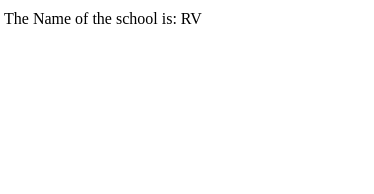# How to select first object in object in AngularJS?

The main problem that we are dealing with is that for an object of objects reading the object of a particular index position is not as simple as a list. We cannot loop over it using ngFor as an object is not considered an iterable. The importance of this issue may arise when the data received from any source is an object containing objects(like JSON files). Altering the source file is very inconvenient so we need to be able to do something in the application itself. The most effective way is hidden in the problem itself. If objects are not iterable, then convert them to iterable.

The following are 2 approaches that can be utilized to select the first object in object in AngularJS, which is described below:

## Approach 1: Using the ng-repeat Directive and the limitTo Filter

• The ng-repeat is able to iterate over the properties of objects which in our case are objects themselves. The following syntax is used:
`<div ng-repeat="(key, value) in myObj">     ... </div>`
• limitTo filter creates a new array or string containing only a specified number of elements. The elements are taken from either the beginning or the end of the source array, string, or number, as specified by the value and sign (positive or negative) of the limit. The following syntax will be used to bind the HTML:
`{{ limitTo_expression | limitTo : limit : begin}}`

Example 1: In this example, the value of the limit can be changed to get the element(object in this case) of the iterable.

## HTML

 `` `<``html``>`   `<``head``>` `    ``<``title``>` `        ``Angular first object in object` `    ``` `    ``<``script` `src``=` `"https://ajax.googleapis.com/ajax/libs/angularjs/1.6.9/angular.min.js"``>` `    ``` ``   `<``body` `ng-app``=``'MyApp'` `      ``ng-controller``=``'MyCtrl'``>` `    ``<``div` `ng-repeat``=``"(key, obj) in institute | limitTo : 1"``>` `        ``<``ul``>` `            ``<``li` `ng-repeat``=``"(prop, value) in obj"``>` `                ``{{prop}}: {{value}}` `            ``` `        ``` `    ``` `    `  `    ``<``script``>` `        ``var app = angular.module('MyApp', []);` `        ``app.controller('MyCtrl', function(\$scope) {` `            ``\$scope.institute = {` `                ``'school': {` `                    ``location: 'Jamshedpur',` `                    ``name: 'RV'` `                ``},` `                ``'college': {` `                    ``location: 'Kolkata',` `                    ``name: 'Jadavpur'` `                ``}` `            ``};` `            ``var instilist = new Array();` `            ``for (key of Object.keys(\$scope.institute)) {` `                ``instilist.push(\$scope.institute[key]);` `            ``}` `            ``\$scope.institute = instilist;` `        ``});` `    ``` ``   ``

Output:## Approach 2

Convert the object of objects into an array of objects and use the indexing method of square brackets([]) to display the first object of the array.

Syntax:

`{{name_of_the array[index]}}`

Example 2: In this example, the value of the index is 0 for the first object of the array. Since the elements of the array are objects we can refer to the properties of the objects using the (.) operator.

## HTML

 `` `<``html``>`   `<``head``>` `    ``<``title``>` `        ``Angular first object in object` `    ``` `    ``<``script` `src``=` `"https://ajax.googleapis.com/ajax/libs/angularjs/1.3.14/angular.min.js"``>` `    ``` ``   `<``body``>` `    ``<``div` `ng-app``=``"MyApp"` `         ``ng-controller``=``"MyCtrl"``>` `        ``<``p``>` `            ``The Name of the school is: {{institute.name}}` `        ``` `    ```   `    ``<``script``>` `        ``var app = angular.module('MyApp', []);` `        ``app.controller('MyCtrl', function(\$scope) {` `            ``\$scope.institute = {` `                ``school: {` `                    ``location: 'Jamshedpur',` `                    ``name: 'RV'` `                ``},` `                ``college: {` `                    ``location: 'Kolkata',` `                    ``name: 'Jadavpur'` `                ``}` `            ``};` `            ``var instilist = new Array();` `            ``for (key of Object.keys(\$scope.institute)) {` `                ``instilist.push(\$scope.institute[key]);` `            ``}` `            ``\$scope.institute = instilist;` `        ``});` `    ``` ``   ``

Output:Whether you're preparing for your first job interview or aiming to upskill in this ever-evolving tech landscape, GeeksforGeeks Courses are your key to success. We provide top-quality content at affordable prices, all geared towards accelerating your growth in a time-bound manner. Join the millions we've already empowered, and we're here to do the same for you. Don't miss out - check it out now!

Previous
Next